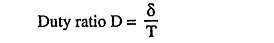## Pulse Signals Interview Questions and Answers:

1. Define periodic signal.

Ans. Periodic signal is that which repeats itself at regular intervals of time.

2. What is meant by a time period of a periodic signal?

Ans. Time period of a periodic signal is defined as the time duration in seconds between the start of one pulse and the start of the next pulse.

3. What is the relationship between time period T and repetition frequency, fR?

Ans. fR = 1/T

4. What is meant by duty ratio? Give relationship between ‘ON period’ δ, pulse repetition period T and duty ratio.

Ans. Duty ratio is defined as the ratio of the ‘ON’ period δ to the pulse repetition period T and is denoted by symbol D.5. Define aperiodic signal and random signal.

Ans. Aperiodic signal is that which occurs only once in the entire time period. Transient of different forms constitute such signals. Random signal is that which occurs without any well defined mathematical description. Noise is one such signal.

6. Define rise time and decay time of a wave.

Ans. The time the wave takes to increase from 10 per cent to 90 per cent of its normal amplitude is called the rise time of the wave. The time the wave takes to decrease from 90 per cent to 10 per cent of its normal amplitude is called the decay time of the wave.

7. What do you mean by resolving time and settling time of circuit?

Ans. Resolving time is defined as the time required by the circuit to provide its first output.

Settling time is defined as the time required by the circuit output to settle down to its final steady-state position after the application of the input.

8. How do square-wave generators differ from pulse generators?

Ans. Square-wave generators can be considered to be a special class of pulse generators. If the pulse train has the property of being “on” 50% of the time period and “off” 50% of the time period, the waveform generated is called the square wave and the generators producing such waveforms are called the square-wave generators.

9. What is is waveforming?

Ans. Waveforming is a process of generating new waveshapes from older waveshapes by using some network.

10. Give some examples of linear and nonlinear waveshaping circuits.

Ans. Linear waveshaping circuits include R-C, R-L, R-L-C circuits whereas nonlinear waveshaping circuits include clipping and clamping circuits.

Scroll to Top